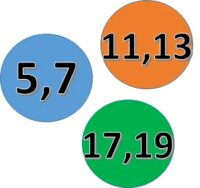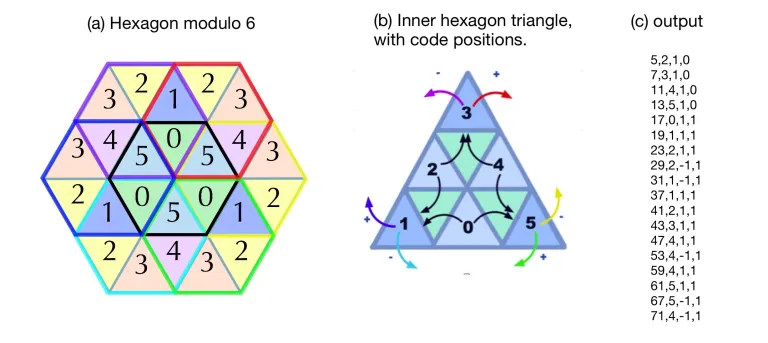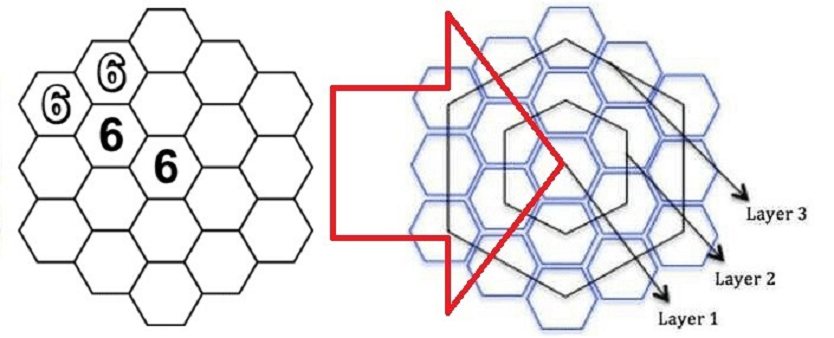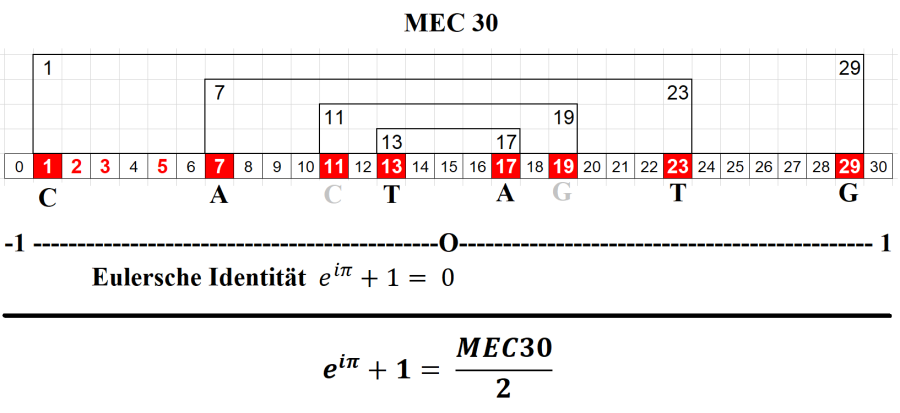# True Prime Pairs

This's the partial of the mapping scheme of our ₠Quantum Project. In short this project is mapping the quantum way within a huge of prime objects (5 to 19) by lexering (11) the ungrammared feed (7) and parsering (13) across syntax (17).The 5+7+11+13 is the smallest square number expressible as the sum of four consecutive primes which are also two couples of prime twins! Their sum is 36 which is the smallest square that is the sum of a twin prime pair {17, 19}

``````\$True Prime Pairs:
(5,7), (11,13), (17,19)

layer|  i  |   f
-----+-----+---------
|  1  | 5
1  +-----+
|  2  | 7
-----+-----+---  } 36 » 6®
|  3  | 11
2  +-----+
|  4  | 13
-----+-----+---------
|  5  | 17
3  +-----+     } 36 » 6®
|  6  | 19
-----+-----+---------
``````

This 36 is the smallest number expressible as the sum of consecutive prime in two (2) ways (5+7+11+13 and 17+19). Our mapping is simulating a recombination of the three (3) layers of this prime pair. See how these layers will behave there:

Note

This progression 41,43,47,53,61,71,83,97,113,131 whose general term is 41+x+xx, is as much remarkable since the 40 first terms are all prime numbers (Euler’s letter to Bernoulli).

``````1st layer:
It has a total of 1000 numbers
Total primes = π(1000) = 168 primes

2nd layer:
It will start by π(168)+1 as the 40th prime
It has 100x100 numbers or π(π(10000)) = 201 primes
Total cum primes = 168 + (201-40) = 168+161 = 329 primes

3rd layer:
Behave reversal to 2nd layer which has a total of 329 primes
The primes will start by π(π(π(1000th prime)))+1 as the 40th prime
This 1000 primes will become 1000 numbers by 1st layer of the next level
Total of all primes = 329 + (329-40) = 329+289 = 618 = 619-1 = 619 primes - Δ1
``````

You may refer to the structure of minor hexagon it shows that this reversal behaviour is linked to the nature of the prime numbers. More interesting is that, like the Prime Hexagon it self, they are newly discovered.

## Reversal behaviour

Note

Aside from 2 and 3, primes come in two flavors, 1 modulo 6 and 5 modulo 6, or the dark and light blue triangles in figure 2(a). The program determines where primes land in the hexagon by moving between the 6 possible positions where primes may land, figure 2(b) . The 1-type primes land in python cells 1, 3, and 5. The 5-type primes land in 0, 2, and 4 cells. Finally, it can print output in the form of figure 2(c). (HexSpin)The 619 is the 114th prime. By the True Prime Pairs it is laid on the last index of 6 with prime 19 where as 6x19 is also 114. Let's put 19 hexagons within the 3 layers.

Tip

When we multiply 114 minor hexagons again by six (6) minus the duplicaton within those layers we got 114x6-30-30-5 = 684-65 which is also 619. So what. Are these 30,30, 5 also related with (Δ1)?There are many other prime curiousity has been stated for this number 619 but almost none about 619-1 which is 618. So here we are going to discuss about this number particularly with the said recombination which resulting the above Δ1 with 619.

Note

TON *618* is the largest black hole in the universe. It’s so large that it has pioneered the classification of “Ultramassive black hole,” with Solar Mass of 66 trillion of our suns! Boasts an extremely high gravitational pull as a result of inspiring mass, and might have been formed by the merging of more than one black hole in the past (Largest.org).

## Section layers

The avove scheme is also applied in to our project sections which is consists of four (4) zones, the first layer covers addition and miltiplication zones, the rest are covered by the second and third layers.

This scheme is actually the quantum way of our project algorithm. This way will also be our approach to Euler's identity showing two distinc halves of (Δ1) from middle 0 in The Primes Platform.Let's discuss about where this (Δ1) is actually coming from.